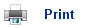# Redox Reactions

• Oxidation can be described as a reaction in which hydrogen is lost or oxygen is gained.
• Reduction can be defined as a reaction in which hydrogen is gained or oxygen is lost.

For example, CuO(s) + H2(g)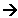Cu(s) + H2O(l)

• In the above reaction copper oxide loses oxygen, so it has been reduced. Hydrogen gains oxygen so it has been oxidised.
• Oxidation and Reduction always occur together in what is called a redox reaction.

## Redox in terms of Electron Transfer

• Redox reactions can also be described in terms of electron transfers.
• Reduction is the gain of electrons, and oxidation is the loss of electrons.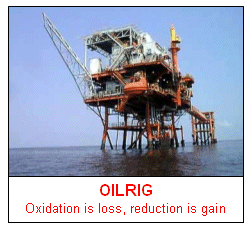Consider the following reaction:

Mg(s) + Cl2(g)MgCl2(s)

• The above reaction shows the reaction between Magnesium and Chlorine.
• There are two processes occurring in the reaction above:
1. Oxidation reaction:     The magnesium is losing electrons:

MgMg2+ + 2e-

2. Reduction reaction:     The chlorine is gaining electrons:

Cl2 + 2e-2Cl-
• These two equations are called half equations.
• The reducing agent is an electron donor, in the previous reaction it is the Magnesium.
• The oxidising agent is an electron acceptor (thief), in the previous reaction it is the Chlorine.

## Oxidation Numbers

• Oxidation numbers are assigned to an atom or an ion to describe its relative state of oxidation or reduction. Using the oxidation numbers, it is possible to deduce whether a redox reaction has occurred and to identify the oxidising agent and reducing agent.
• Rules for assigning Oxidation numbers: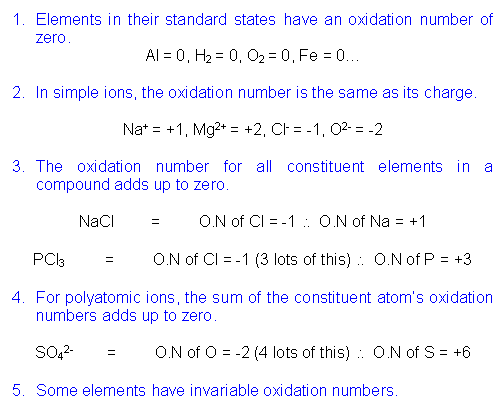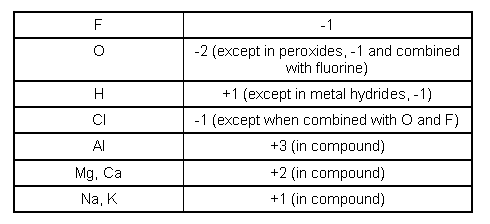• If the oxidation number increases, the element is oxidised.
• If the oxidation number decreases, the element is reduced.
• The total change in an oxidation number increasing and decreasing in a reaction, must be the same in both directions.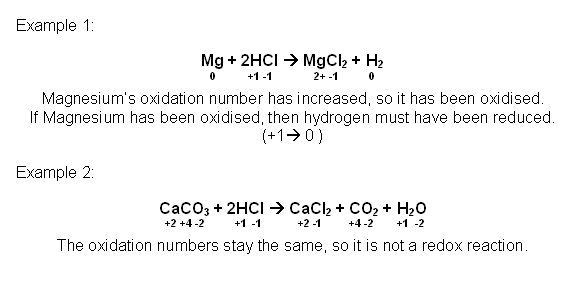## Useful books for revision:

Revise AS Chemistry for Salters (Written by experienced examiners and teachers of Salter's chemistry)Revise AS Chemistry for Salters (OCR) (Salters Advanced Chemistry)Home